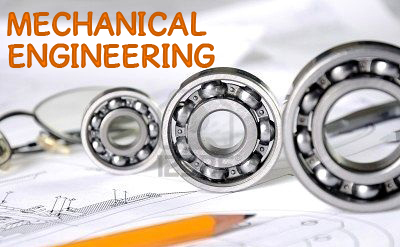Basic mechanics is, in the most general sense, the study of forces and their effect upon matter. Typically, engineering mechanics is used to analyze and predict the acceleration and deformation (both elastic and plastic) of objects under known forces (also called loads) or stresses. Subdisciplines of mechanics include:• Statics, the study of non-moving bodies under known loads, how forces affect static bodies
• Dynamics (or kinetics), the study of how forces affect moving bodies
• Mechanics of materials, the study of how different materials deform under various types of stress
• Fluid mechanics, the study of how fluids react to forces
• Kinematics, the study of the motion of bodies (objects) and systems (groups of objects), while ignoring the forces that cause the motion. Kinematics is often used in the design and analysis of mechanisms.
• Continuum mechanics, a method of applying mechanics that assumes that objects are continuous (rather than discrete)

Here is the list of Basic Mechanical Engineering Practicals –

• ## To conduct Impact Test on Impact testing machine

Do check Out – Engineering Workshop Practicals